# 期末复习四——串、数组、广义表

### 串

#### BF匹配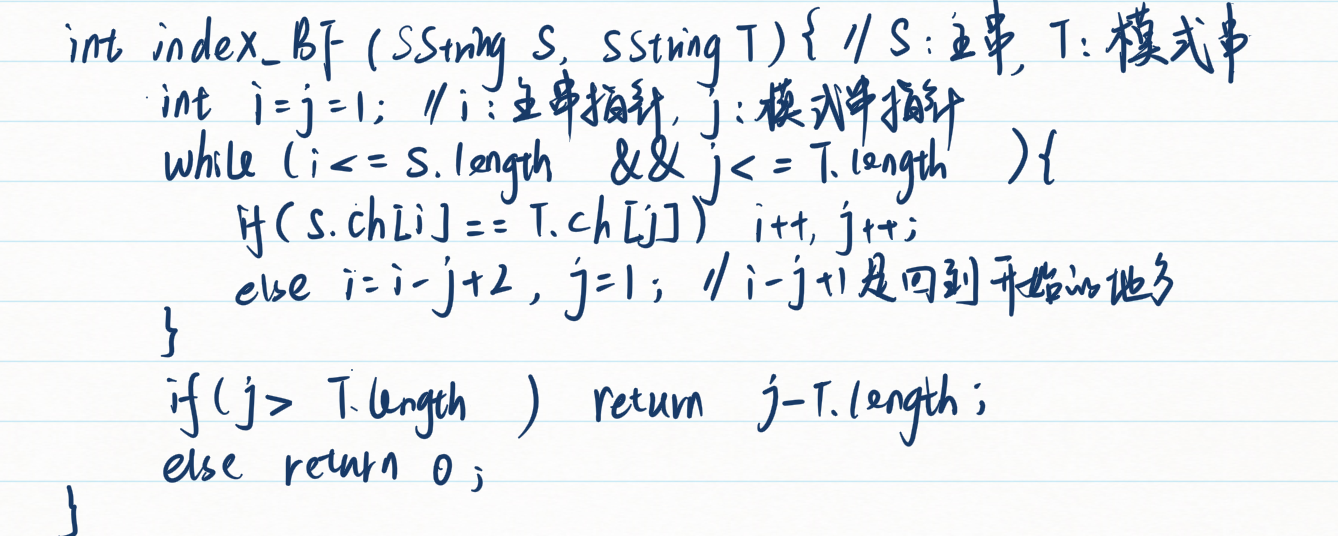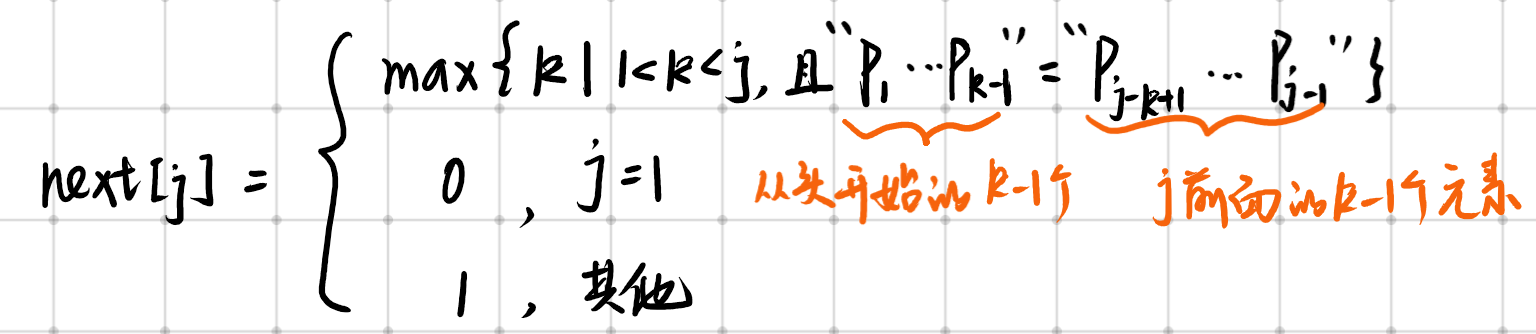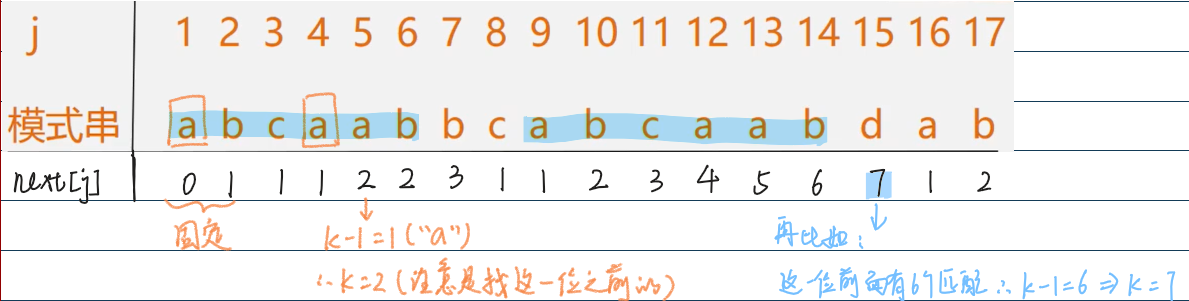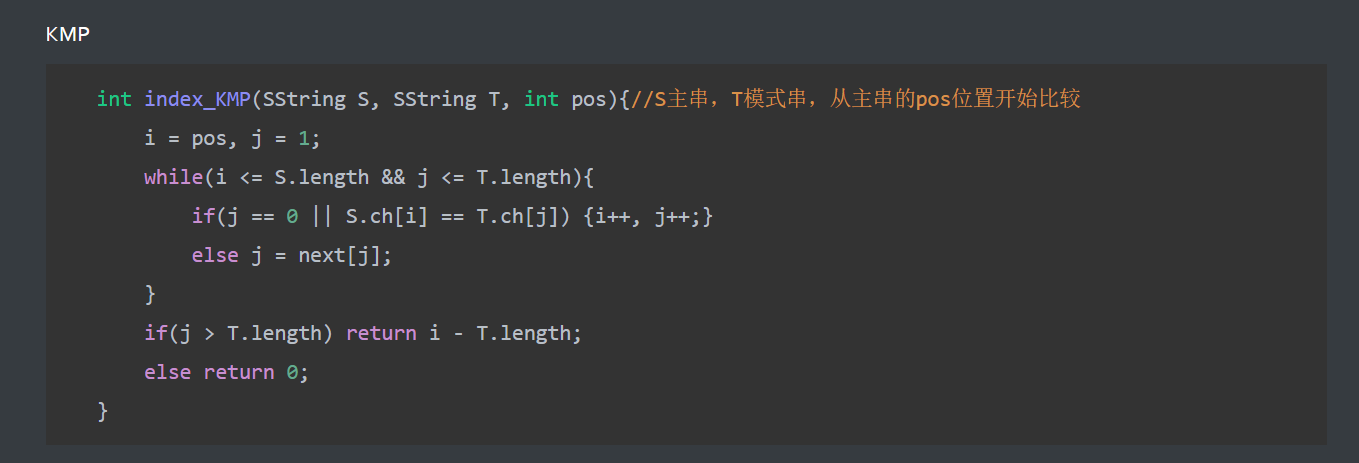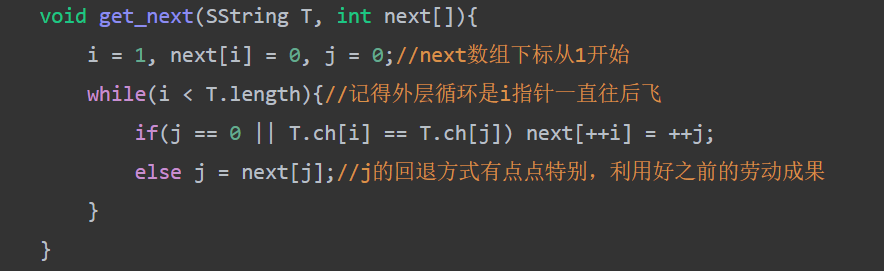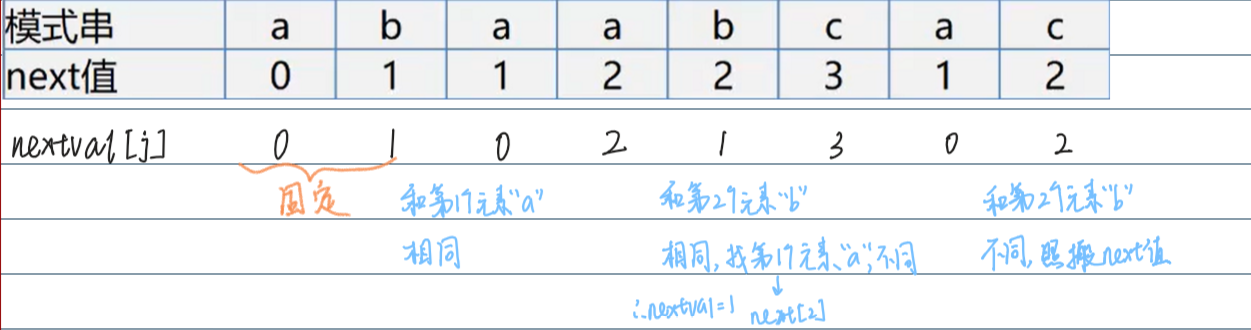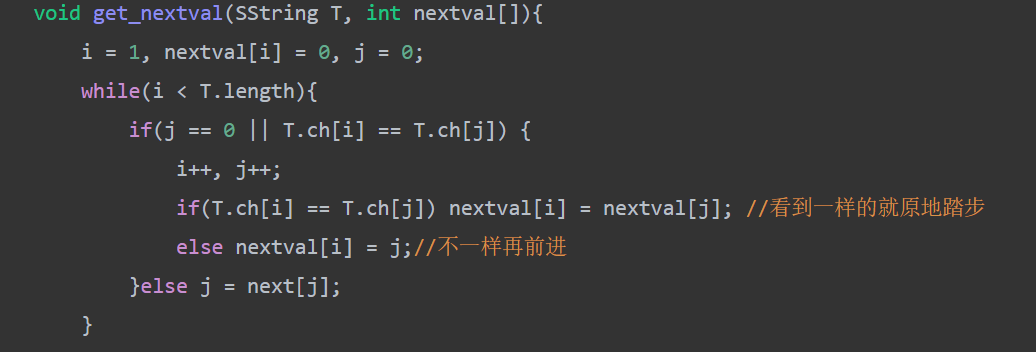### 错题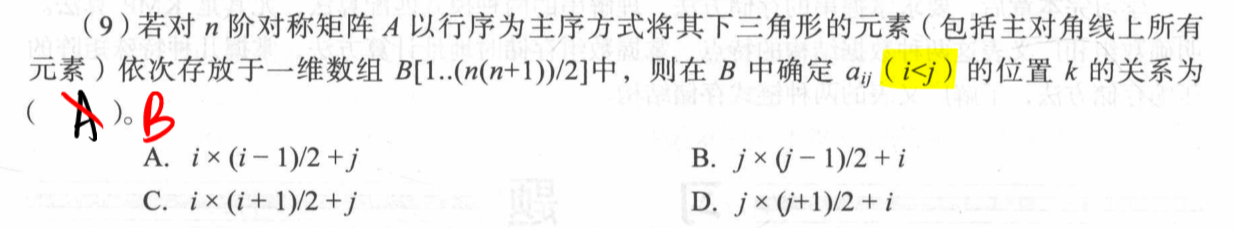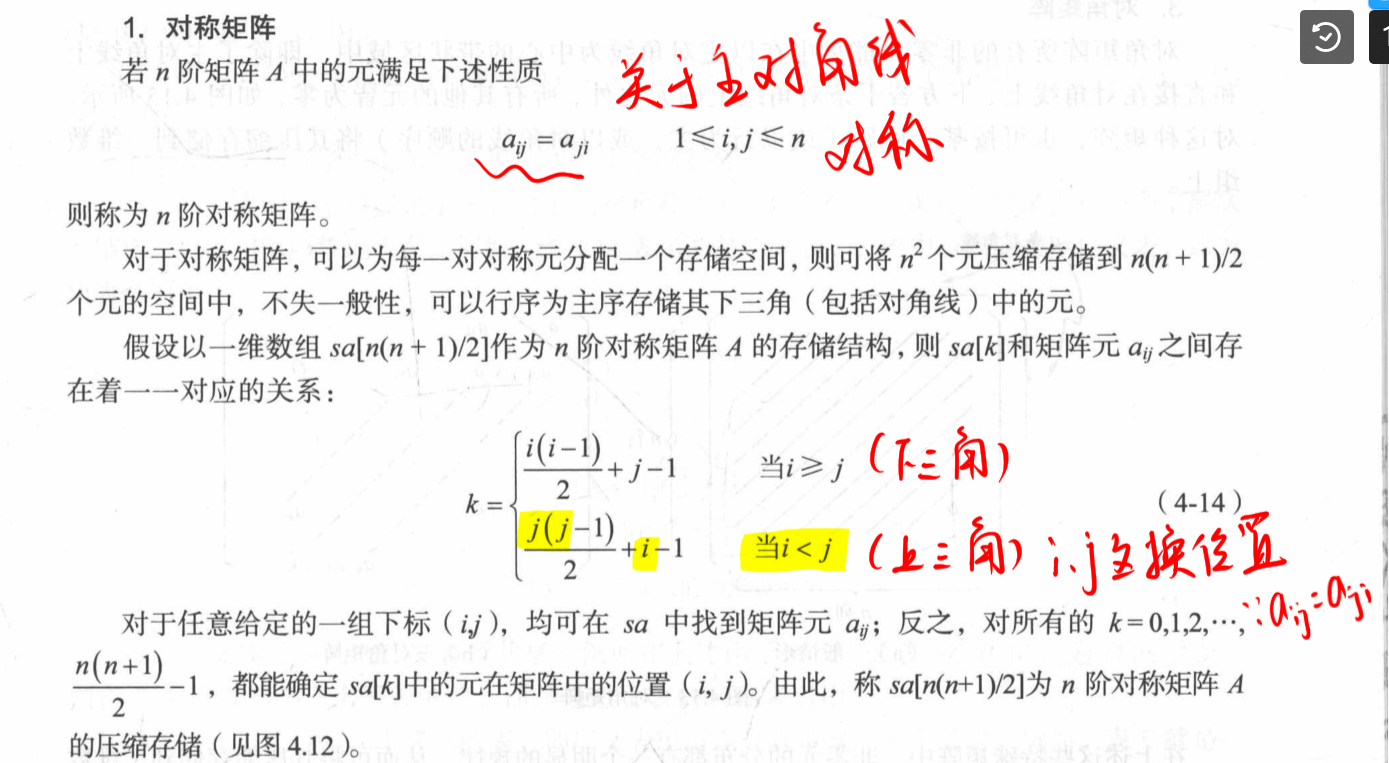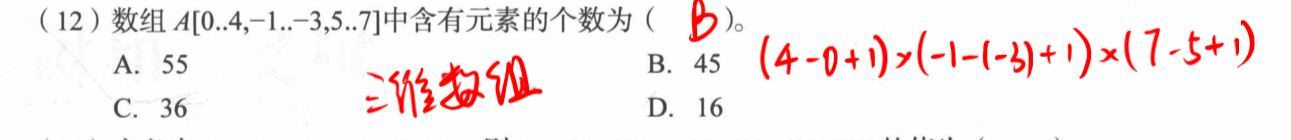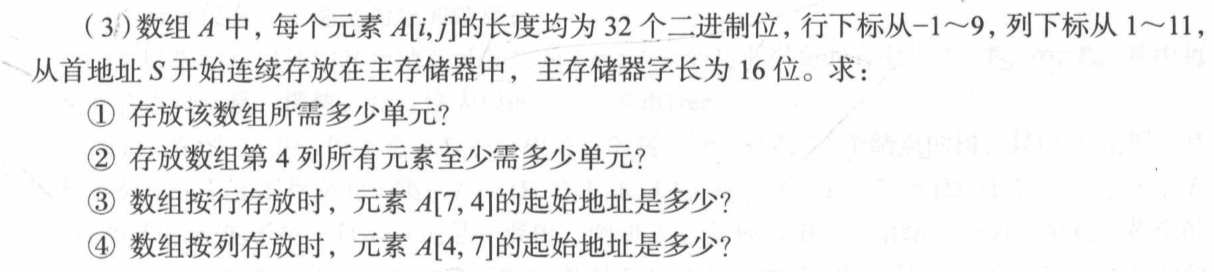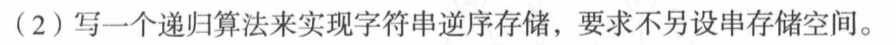好题实现字符串的逆置并不难，但本题“要求不另设串存储空间”来实现字符串逆序存储，即第一个输入的字符最后存储，最后输入的字符先存储，使用递归可容易做到。

void InvertStore(char A[])
//字符串逆序存储的递归算法。
{
char ch;
static int i = 0;//需要使用静态变量
cin>>ch;
if (ch!= ‘.’) //规定’.'是字符串输入结束标志
{
InvertStore(A);
A[i++] = ch;//字符串逆序存储，操作放在递归的后面
}
A[i] = ‘\0’; //字符串结尾标记
}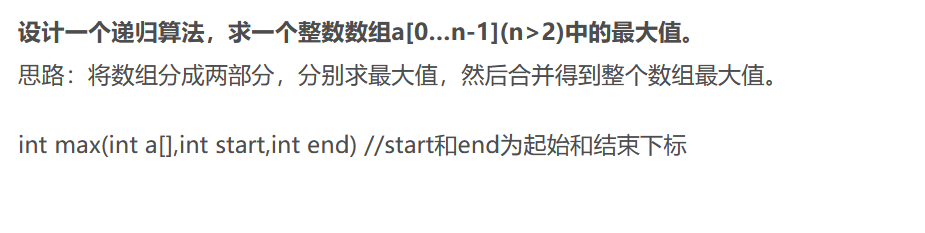int max(int a[],int start, int end){
if(start==end) return a[start]; //出口
else{
mid = (start+end)/2; //取个中
m = max(a,start,mid); //左边喜刷刷
n = max(a,mid+1,end); //右边喜刷刷
return m>n?m:n; //求个大
}
}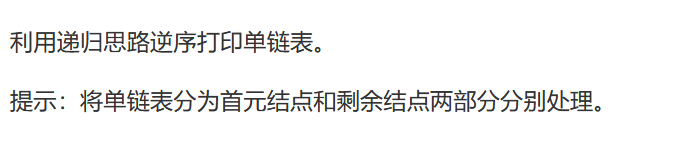void reverse_print(LinkList head){
return;
else{
}
}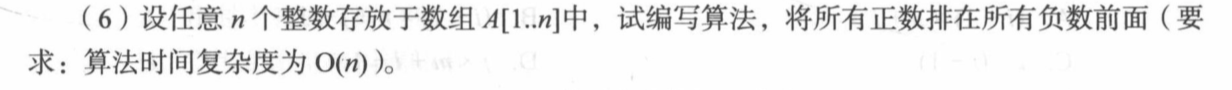void Arrange(int A[],int n)
//n个整数存于数组A中，本算法将数组中所有正数排在所有负数的前面
{
int i=0,j=n-1,x; //用类C编写，数组下标从0开始
while(i<j)
{
while(i<j && A[i]>0) i++;//停在负数上
while(i<j && A[j]<0) j–;//停在正数上
if(i<j) {x=A[i]; A[i++]=A[j]; A[j--]=x; }//交换A[i] 与A[j]
}
}01-055464
12-3013306-02348
02-25846
12-1781
06-232147
08-021009
01-0777
07-3036
12-30344